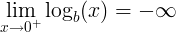Logarithm rules

Logarithm Rules and Properties

Rule name Rule
Logarithm product rule

logb(x ∙ y) = logb(x) + logb(y)

Logarithm quotient rule

logb(x / y) = logb(x) - logb(y)

Logarithm power rule

logb(x y) = y ∙ logb(x)

Logarithm base switch rule

logb(c) = 1 / logc(b)

Logarithm base change rule

logb(x) = logc(x) / logc(b)

Derivative of logarithm

f (x) = logb(x) f ' (x) = 1 / ( x ln(b) )

Integral of logarithm

logb(x) dx = x ∙ ( logb(x) - 1 / ln(b) ) + C

Logarithm of 0

logb(0) is undefinedLogarithm of 1

logb(1) = 0

Logarithm of the base

logb(b) = 1

Logarithm of infinity

lim logb(x) = ∞, when x→∞

Logarithm product rule

The logarithm of a multiplication of x and y is the sum of logarithm of x and logarithm of y.

logb(x ∙ y) = logb(x) + logb(y)

For example:

logb(37) = logb(3) + logb(7)

The product rule can be used for fast multiplication calculation using addition operation.

The product of x multiplied by y is the inverse logarithm of the sum of logb(x) and logb(y):

x ∙ y = log-1(logb(x) + logb(y))

Logarithm quotient rule

The logarithm of a division of x and y is the difference of logarithm of x and logarithm of y.

logb(x / y) = logb(x) - logb(y)

For example:

logb(3 / 7) = logb(3) - logb(7)

The quotient rule can be used for fast division calculation using subtraction operation.

The quotient of x divided by y is the inverse logarithm of the subtraction of logb(x) and logb(y):

x / y = log-1(logb(x) - logb(y))

Logarithm power rule

The logarithm of the exponent of x raised to the power of y, is y times the logarithm of x.

logb(x y) = y ∙ logb(x)

For example:

logb(28) = 8logb(2)

The power rule can be used for fast exponent calculation using multiplication operation.

The exponent of x raised to the power of y is equal to the inverse logarithm of the multiplication of y and logb(x):

x y = log-1(y ∙ logb(x))

Logarithm base switch

The base b logarithm of c is 1 divided by the base c logarithm of b.

logb(c) = 1 / logc(b)

For example:

log2(8) = 1 / log8(2)

Logarithm base change

The base b logarithm of x is base c logarithm of x divided by the base c logarithm of b.

logb(x) = logc(x) / logc(b)

Logarithm of 0

The base b logarithm of zero is undefined:

logb(0) is undefined

The limit near 0 is minus infinity:Logarithm of 1

The base b logarithm of one is zero:

logb(1) = 0

log2(1) = 0

Logarithm of the base

The base b logarithm of b is one:

logb(b) = 1

log2(2) = 1

Logarithm derivative

When

f (x) = logb(x)

Then the derivative of f(x):

f ' (x) = 1 / ( x ln(b) )

For example:

When

f (x) = log2(x)

Then the derivative of f(x):

f ' (x) = 1 / ( x ln(2) )

Logarithm integral

The integral of logarithm of x:

logb(x) dx = x ∙ ( logb(x) - 1 / ln(b) ) + C

For example:

log2(x) dx = x ∙ ( log2(x) - 1 / ln(2) ) + C

Logarithm approximation

log2(x) ≈ n + (x/2n - 1) ,

Logarithm of zero »

Currently, we have around 1977 calculators, conversion tables and usefull online tools and software features for students, teaching and teachers, designers and simply for everyone.